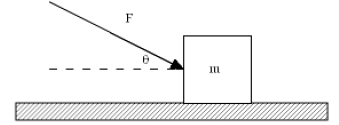## Tuesday, October 13, 2015

### A block with mass m is being pushed by a constant force F that makes an angle of θ with the horizontal

A block with mass m is being pushed by a constant force F that makes an angle of θ with the horizontal as shown below. The block is moving with constant velocity on a level surface. The coefficient of kinetic friction between the block and the surface is µk. Which one of the following equations is correct for the magnitude of F?Solution
Let |F| is the magnitude of the force F
Given Data: m, F, θ, a=0, µk
|F| = |F(m, θ, µk)|=?

FNET=0 because a=0
X direction:   |F|·cos θ + f = FNET, X = 0
Y direction: N mg –  |F|·sin θ = FNET, Y = 0,
where N is the normal force,
g is the gravity acceleration
f= µk N
|F|·cos θ + µk (mg- |F|·sin θ) = 0
|F|·cos θ + µk mg - µk |F|·sin θ = 0
|F|·cos θ  - µk |F|·sin θ = µk mg
|F|·( cos θ  - µk sin θ) = µk mg

|F| = µk mg/( cos θ  - µk sin θ)

#### Post a Comment

http://tutorstate.blogspot.com/